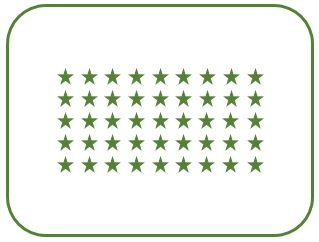# C program to print rectangular star pattern

• Write a program in C to print rectangular star (*) pattern of n rows and m columns using for loop.
• Write a C program to print a rectangle pattern of star (*) character using loops.
For a rectangular star pattern of 5 rows and 9 columns. Program's output should be:

### RECTANGLE STAR PATTERN#### Required Knowledge

Algorithm to print rectangular star pattern using loop
• Take the number of rows(N) and columns(M) of rectangle as input from user using scanf function.
• We will use two for loops to print rectangular star pattern.
• Outer for loop will iterate N times. In each iteration, it will print one row of pattern.
• Inner for loop will iterate M times.In one iteration, it will print one star (*) characters in a row.

## C program to print rectangular star pattern

```#include<stdio.h>

int main(){
int rows, cols , i, j;

printf("Enter rows and columns of rectangle\n");
scanf("%d %d", &rows, &cols);

/* Row iterator for loop */
for(i = 0; i < rows; i++){
/* Column iterator for loop */
for(j = 0; j < cols; j++){
printf("*");
}
printf("\n");
}
return 0;
}
```
Output
```Enter rows and columns of rectangle
3 9
*********
*********
*********
```

Related Topics
 C program hollow rectangle pattern C program square star pattern C program pyramid star pattern C program rhombus star pattern C program diamond star pattern C program binary rectangle pattern C program heart shape star pattern C program palindrome triangle pattern C program prime number triangle pattern List of all C pattern programs